### CAT Number System Tricks (advanced level): Finding Last Non-Zero Digit of any factorial

This article is written by a 100-percentiler in CAT 2016. He is a director of a leading test-prep institute and is the best person to learn Mathematics from. In fact, he was the trainer for Wordpandit himself and Wordpandit credits a lot of his learning to this Master Trainer. Dig into this amazing article by this Mathematics Wizard. We will reveal his identity in the coming posts..:)

Let’s get started with this Advanced maths blog on this topic.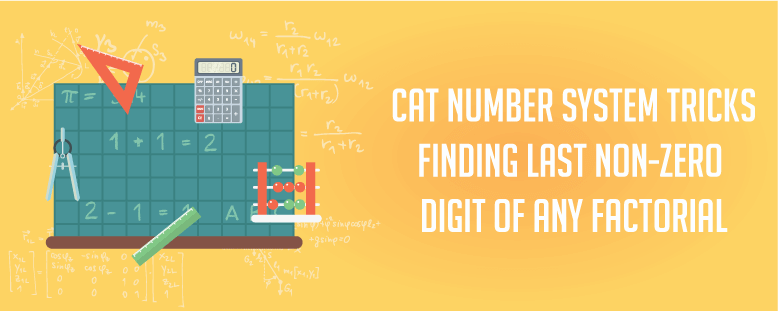Suppose we have to find last non-zero digit of a simple number say 98670000. Obviously, everyone knows the answer is 7. Another perspective to look at it is if we pull all zeros out of the given number then unit digit of remnant (remaining part) will be our answer i.e. we can write
98670000= 10000*(9867)
And unit digit of 9867 is 7
Let us try to apply similar sort of approach to some problems involving factorial.

Question 1: Find last non-zero digit of 18!?
Solution: We will try to solve this problem by first pulling the number of zeros out of given number 18! and then calculating the unit digit of remnant. So we first calculate the number of zeros in 18!.
We know 18!=1×2× 3×4×5×6×7×8×9×10×11×12×13×14×15×16×17×18
Clearly, calculating number of zeros is equivalent to calculating number of 2’s and 5’s
Now we first calculate number of 2’s present in 18! (Maximum power of 2 present in 18!).For that we will move stepwise

Step 1
We first count those numbers which can contribute 2.Clearly we have 9 such numbers i.e. all evens- 2,4,6,8,10,12,14,16,18.We take 21 from each of these numbers i.e. collectively we will have 29 as each of the even number contributed power of 2.

Step 2
Out of the remaining numbers after step 1, again we count those numbers that can contribute 2.
Clearly after step 1 i.e. after pulling 2 from all even numbers we are left with  1,2,3,4,5,6,7,8,9.Out of these, we count those that can contribute 2. We have 4 such numbers i.e. all evens- 2,4,6,8.We take 21 from each of these numbers i.e. collectively we will have 24 as each of the even number contributed power of 2.

Step 3
Out of the remaining numbers after step 2 again we count those numbers that can contribute 2.
Clearly after step 2 i.e. after pulling 2 from all remaining even numbers we are left with  1,2,3,4.Out of these,we count those that now can contribute 2.We have 2 such numbers i.e. all evens- 2 and 4.We take 21 from each of these numbers i.e. collectively we will have 22 as each of the even number contributed power of 2.

Step 4
Out of the remaining numbers after step 3 again we count those numbers that can contribute 2.
Clearly, after step 3 i.e. after pulling 2 from all remaining even numbers we are left with 1 and 2.Out of these, we count those that now can contribute 2.Clearly, we have only have 1 such number.
Adding all four steps we can say maximum power of 2 present in 18! =9+4+2+1=16
In fact we can generalize what we did above for calculating maximum power of any prime p in any n!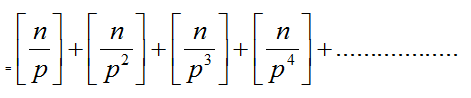Where[ ] represents integral part
So for calculating maximum power of 5 in 18! We apply the above formula directly
Maximum power of 5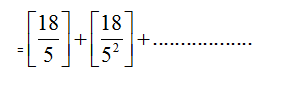= 3+ 0

=3
So, the number of zeros present in 18! will be Minimum of (3,16)=3(Because for making power of 10 we require both 2 and 5 thus minimum power present will determine the number of zeros)
In fact, we can write prime factorization of 18! by calculating maximum power of each prime involved

Maximum power of 3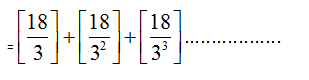= 6+2
=8
Maximum power of 7 =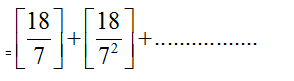= 2+ 0
=2
Clearly,
Maximum power of 11 = 1
Maximum power of 13 = 1
Maximum power of 17 = 1

Thus prime factorization of 18! is as follows
18! = 2^16. 3^8. 5^3. 7^2. 11^1. 13^1.17^1
So we pull all zeros out of the given number then calculate the unit digit of remnant (remaining part)
2^3. 5^3(2^13. 3^8. 7^2. 11^1. 13^1.17^1)

Now to calculate Unit digit of 2^13. 3^8. 7^2. 11^1. 13^1.17^1, we calculate unit digit of each separately.
Unit digit of 2^13=2
Unit digit of 3^8=1
Unit digit of 7^2=9
Unit digit of 13=3
Unit digit of 17=7
Unit digit of 2^13. 3^8. 7^2. 11^1. 13^1.17^1=2×1×9×1×3×7=8

NOTE: The question we solved was easy as 18! is not a very big number and we were able to factorise it easily. Now the question arises what if the number is big and process of factorization is very lengthy.

### Complete List of Articles in this Series

Question 2: Find last non-zero digit of 2000!?
Solution: We know 2000! is product of 1st 2000 natural numbers. We write these numbers in set of 10 numbers each.
We know
2000! =1x2x3x4x5x6x7x8x9x10x11x12x13x14x15x16x17x18x19x20…………………………x2000
= (1x2x3x4x5x6x7x8x9x10)(11x12x13x14x15x16x17x18x19x20)……………………………………………………………
……………………………………………………………(1991x1992x1993x1994x1995x1996x1997x1998x1999x2000)
i.e. we divided 2000! in groups of 10 each
We write 1st 2000 natural numbers in general form as
10a+1
10a+2
10a+3
10a+4
10a+5
10a+6
10a+7
10a+8
10a+9
10a+10(or we can write it as 10a+0)
Where a varies from 0 to 199 to cover all 1st 2000 natural numbers
When a=0 Then above set of numbers reduces to 1,2,3…….10
Similarly when a=1 Then above set of numbers reduces to 11,12,13…….20
Similarly when a=2 Then above set of numbers reduces to 21,22,23…….30
…………………………………………………………………………………………………………………..
Similarly when a= 199 Then above set of numbers reduces to 1991,1992,1993…….2000
Let us look at the following observations:

Observation 1
(10a+1)(10a+3)(10a+7)(10a+9)
= (10a+1)(10a+9)(10a+3)(10a+7)
=(100a2+100a+9) (100a2+100a+21 )
=10000a4+10000a3+900a2+10000a3+10000a2+900a+2100a2+2100a+189
=10000a4+20000a3+13000a2+3000a+189
=1000(Some integer) +3000a+189
=1000(X) +3000a+189 where X is some integer

Observation 2
(10a+2)(10a+4)(10a+6)(10a+8)
= (10a+2)(10a+8)(10a+4)(10a+6)
= (100a2+100a+16) (100a2+100a+24)
=10000a4+10000a3+1600a2+10000a3+10000a2+1600a+2400a2+2400a+384
=1000(Some integer) +4000a+384
=4 [250(Some integer)+1000a+96]
=22[250(Y)+1000a+96]where Y is some integer

Observation 3
5.10.15.20.25.30.35…………………………………………………………………………..1990.1995.2000
=5400(1.2.3.4.5.6.7………………………………………………………………………………….398.399.400)
=5400(400!)

Let us make use of the above three observations we made in calculating last nonzero digit
Now we pull two 5’s from each group of 10 numbers and making use of Observation 3
We can write
2000!== (1.2.3.4.5.6.7.8.9.10)(11.12.13.14.15.16.17.18.19.20)……………………………………………………………
……………………………………………………………(1991.1992.1993.1994.1995.1996.1997.1998.1999.2000)
=(5.10.15.20.25.30.35………………………….……………….…1990.1995.2000)(1.2.3.4.6.7.8.9) (11.12.13.14.16.17.18.19)………………………………………… (1991.1992.1993.1994.1996.1997.1998.1999)
=5400(400!)(1.2.3.4.6.7.8.9)(11.12.13.14.16.17.18.19)…………………………………………
…………………………………………………………………………(1991.1992.1993.1994.1996.1997.1998.1999) …………….. (A)
Notice that after pulling two 5`s from each group of 10 numbers we have 5400(400!)as shown above and we are left with 8 numbers in each group which can be written in generalised form as
(10a+1)(10a+2)(10a+3)(10a+4)(10a+6)(10a+7)(10a+8)(10a+9)
Putting different values of a, we will get members of each group e.g.
Putting a=0 we get members of first group (1.2.3.4.6.7.8.9)
Putting a=1 we get members of second group (11.12.13.14.16.17.18.19)
……………………………………………………………………………………………………………..
……………………………………………………………………………………………………………..
Putting a= 199 we get members of last group (1991.1992.1993.1994.1996.1997.1998.1999)

Now using Observation 1 and 2 we can write
(10a+1)(10a+2)(10a+3)(10a+4)(10a+6)(10a+7)(10a+8)(10a+9)
= (10a+1)(10a+3)(10a+7)(10a+9)(10a+2)(10a+4)(10a+6)(10a+8)
= [1000(X) +3000a+189][22[250(Y)+1000a+96]]
=22[1000(X) +3000a+189][250(Y)+1000a+96]
We have pulled 22 from group of these 8 numbers and in totality we have 200 such sets.
Each such set can contribute 22 and thus we have total of 2400 outside.
From observation 3 we had 5400 outside. Thus in totality by combining 2400 and 5400 we have (2400)(5400)= 10400 i.e. we pull all 400 zeros out of the given number then unit digit of remnant (remaining part) will be our answer.
So lets us look at unit digit of[1000(X) +3000a+189][250(Y) +1000a+96]
= Unit digit of[1000(X) +3000a+189].Unit digit of[250(Y) +1000a+96]
= 9.6
= 4 ……………………………………………………………. (B)
In 1 set of 10 numbers the unit digit of remaining numbers after pulling 400 zeros is 4.Clearly we have 200 such sets with us and each such set gives 4 as unit digit. Thus, in totality, we will have 4200.Thus Combining (A) and (B) and after pulling 400 zeros we can say

Last non-zero digit of 2000! =Unit Digit after pulling 400 zeroes of (400! 4200)
Thus our question reduces from a big number to relatively small number 400!
Again we follow the same process and we can directly writ
400! = Unit of digit after pulling 80 zeros of (80! 440)
Again we follow the same process and we can directly write
80! = Unit of digit after pulling 16 zeros of (16! 48)
Now we cannot apply same further as we are left with 16!.But 16! Is a very small number and we can easily calculate its unit digit by writing its prime factorization
We know 16! =1.2.3.4.5.6.7.8.9.10.11.12.13.14.15.16+6
Maximum power of 2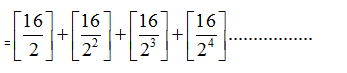= 8+4+ 2 + 1
=15
Maximum power of 3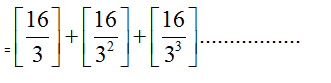= 5+1+0

=6

Maximum power of 5=3
Maximum power of 7=2
Maximum power of 11=1
Maximum power of 13=1
16!=2^15. 3^6. 5^3.7^1. 11^1. 13^1
After pulling zeros we can write it as
16!= 2^3. 5^3(2^12. 3^6. 7^1. 11^1. 13^1)
Thus Unit digit of remnant (remaining part) will be our answer
Unit digit of 2^12=6
Unit digit of 3^6=9
Unit digit of 2^12. 3^6. 7^1. 11^1. 13^1=6.9.7.1.3=4
And Unit digit of 4^8=6
Thus 80! = Unit of digit after pulling zeros of (16! 48)=4×6=4

Question 2: Find last non zero digit of 1000!?
Solution: As we did in last problem we can directly write
1000! =1.2.3.4.5.6.7.8.9.10.11.12.13.14.15.16.17.18.19.20………………………….1000
= (1.2.3.4.5.6.7.8.9.10)(11.12.13.14.15.16.17.18.19.20)……………………………………………………………

……………………………………………………………(991.992.993.994.995.996.997.998.999.1000)
i.e. we divided 1000! In groups of 10 each
We can write
1000!

=(5.10.15.20.25.30.35………………………….……………….…990.995.1000)(1.2.3.4.6.7.8.9) (11.12.13.14.16.17.18.19)………………………………………… (991.992.993.994.996.997.998.999)

=5200(200!)(1.2.3.4.6.7.8.9)(11.12.13.14.16.17.18.19)…………………………………………

…………………………………………………………………………………..(991.992.993.994.996.997.998.999) (A)

Notice that after pulling two 5`s from each group of 10 numbers we are left with 8 numbers ineach group which can be written in generalized form as
(10a+1)(10a+2)(10a+3)(10a+4)(10a+6)(10a+7)(10a+8)(10a+9)

As we did earlier, we have pulled 22 from the group of these 8 numbers. Thus we have total of 2200 outside by pulling 22 from each group of 8 numbers of this product (1.2.3.4.6.7.8.9)(11.12.13.14.16.17.18.19)…………………………………………
…………………………………………………………………………………..(991.992.993.994.996.997.998.999)

Thus in totality by combining 2200 and 5200 we have (2200)(5200)=10200 i.e. we pull all zeroes out of the given number then unit digit of remnant (remaining part) will be our answer.

In 1 set of 10 numbers the unit digit of remaining numbers after pulling zeros is 4.Clearly we have 100 such sets with us.Thus after pulling zeros we can say last non-zero digit of 1000! =Unit Digit after pulling zeros of (200! 4100)
Thus our question reduces from a big number to relatively small number 200!
Again we follow the same process and we can directly write
200! = Unit of digit after pulling zeros out of (40! 420)
Again we can reduce 40! And following the same process, we can write
40! = Unit of digit after pulling zeros out of (8! 44)
Now we cannot apply it further as we are left with 8! which only has single 5 in it. But 8! Is a very small number and we can easily calculate its unit digit by writing its prime factorization
We know 8! =1.2.3.4.5.6.7.8

Maximum power of 2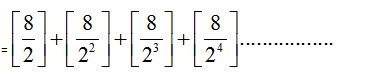= 4+ 2 + 1 +0
=7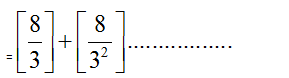= 2+0
=2
Maximum power of 5=1
Maximum power of 7=1
Thus 8! = 2^7.3^2.5^1.7^1
After pulling zeros we can write it as
8! = 2.5(2^6. 3^2.7^1)
Thus Unit digit of remnant (remaining part) will be our answer
Unit digit of 2^6 = 4
Unit digit of 3^2 = 9
Unit digit of 2^6. 3^2. 7^1 = 4.9.7 = 2
And Unit digit of 4^4 = 6
Thus 40! = Unit of digit after pulling zeros out of (8! 44)=2×6=2.

Rest we hope the process is generalised with the help of above three problems and you can calculate last non-zero digit of any factorial.